# 1 Introduction

RaMWAS provides a complete toolset for methylome-wide association studies (MWAS). It is primarily designed for data from enrichment-based methylation assays, but can be applied to other methylomic data (e.g. Illumina methylation array) as well as other data types like gene expression and genotype data (see vignette).

RaMWAS includes the following major components (steps):

1. Scanning aligned reads from BAM files.
2. Calculation of quality control (QC) measures, aggregate QC plots, fragment size distribution estimation.
3. Creation of CpG score (coverage) matrix, sample normalization.
4. Principal component analysis.
5. Methylome-wide association study (MWAS).
6. Annotation of top findings using the BioMart database.
7. Multi-marker analysis (methylation risk score) using elastic net.
8. MWAS with correction for effect of SNPs.

Most steps of RaMWAS are internally parallelized. This is made possible, in part, by the use of the filematrix package for storing the data and accessing it in parallel jobs.

# 2 Getting started

## 2.1 Installation

To install the Bioconductor version of RaMWAS (R 3.4 or newer requred) use the following commands:

## try http:// if https:// URLs are not supported
if (!requireNamespace("BiocManager", quietly=TRUE))
install.packages("BiocManager")
BiocManager::install("ramwas")

The package vignettes and reference manual can be viewed online and with the following commands.

library(ramwas) # Loads the package
browseVignettes("ramwas") # Opens vignettes
help(package = "ramwas") # Lists package functions

# 3 RaMWAS steps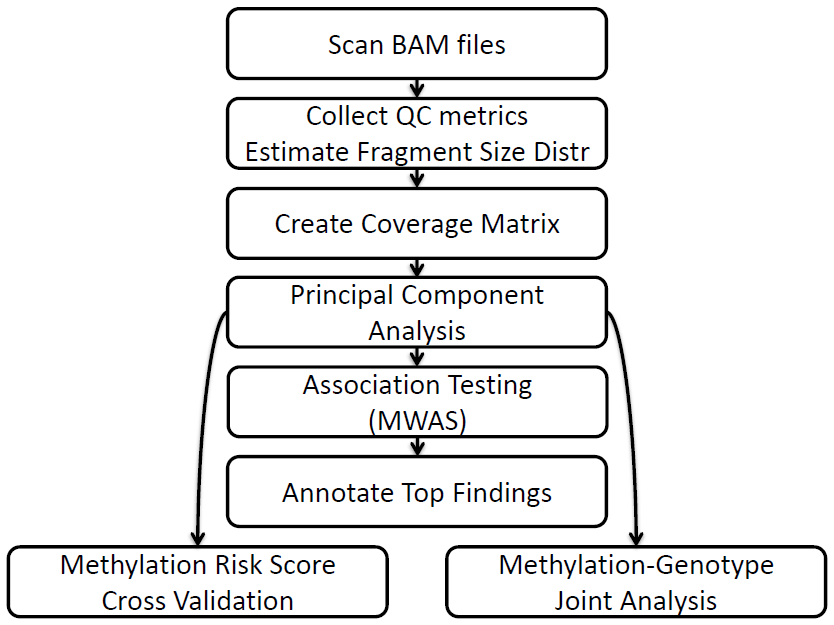Pipeline Workflow

To illustrate the main steps of RaMWAS we first create an artificial data set.

library(ramwas)
dr = paste0(tempdir(), "/simulated_project")
ramwas0createArtificialData(
dir = dr,
nsamples = 20,
ncpgs = 25e3,
cat("Project directory:", dr)
## Project directory: /tmp/RtmpwHjGHT/simulated_project

Note. The project directory dr can be set to a more convenient location when running the code.

The function ramwas0createArtificialData created the following files and subdirectories in the project directory.

• bams – directory with 20 BAM files
• bam_list.txt – file with names of all the BAM files
• covariates.txt – file with age and sex variables for the samples.
The methylation level of some CpGs depends on these covariates.
• Simulated_chromosome.rds - file with genomic locations for all CpGs.

Each RaMWAS step accepts parameters in the form of a list. Here is the parameter set we will use for all steps below.

param = ramwasParameters(
dirproject = dr,
dirbam = "bams",
filebamlist = "bam_list.txt",
filecpgset = "Simulated_chromosome.rds",
scoretag = "MAPQ",
minscore = 4,
minfragmentsize = 50,
maxfragmentsize = 250,
minavgcpgcoverage = 0.3,
minnonzerosamples = 0.3,
filecovariates = "covariates.txt",
modelcovariates = NULL,
modeloutcome = "age",
modelPCs = 0,
toppvthreshold = 1e-5,
bihost = "grch37.ensembl.org",
bimart = "ENSEMBL_MART_ENSEMBL",
bidataset = "hsapiens_gene_ensembl",
biattributes = c("hgnc_symbol","entrezgene","strand"),
bifilters = list(with_hgnc_trans_name = TRUE),
biflank = 0,
cvnfolds = 5,
mmalpha = 0,
mmncpgs = c(5, 10, 50, 100, 500, 1000, 2000)
)

We describe the role of each parameter as they are used below. The complete description of all RaMWAS parameters is available in the parameter vignette.

## 3.1 Scan BAM files and calculate QC indices

This step scans all BAM files listed in the filebamlist file, it records read start locations, and calculates a number of quality control metrics. The BAMs must be located in dirbam directory.

Reads are filtered by the scoretag parameter, which is usually either the “MAPQ” field or “AS” tag in the BAM file (see BAM file format). Reads with scores below minscore are excluded.

The minfragmentsize and maxfragmentsize parameters define the minimum and maximum size of DNA fragments that were sequenced. Please note, these parameters are not equal to the read length but instead reflect the length of the DNA fragments that were extracted and sequenced.

The set of CpGs is loaded from the filecpgset file. For more information see the CpG set vignette.

RaMWAS uses cputhreads CPU cores (parallel jobs) to scan BAMs. Hard disk speed can be a bottleneck for this step. If BAMs are stored on a single rotational hard drive, using more than 4 parallel jobs may not provide further speed improvements.

ramwas1scanBams(param)

This creates the following subdirectories in the project directory:

• qc – directory with a number of quality control (QC) plots, one plot per QC metrix per BAM.
• edit_distance – plots showing distribution of edit distance, i.e. number of mismatches between the aligned read and the reference genome.
• isolated_distance – plots showing distribution of distances from read starts to isolated CpGs.
The CpGs are called isolated if they are located at least maxfragmentsize basepairs away from any other CpG.
The distribution of reads around these CpGs is used later for estimation of fragment size distribution.
• matched_length – plots showing distribution of the length of the reads aligned to the reference genome.
• score – plots showing distribution of the score (scoretag parameter).
• coverage_by_density – plots showing average CpG score (coverage) as a function of CpG density.
These plots illustrate the performance of the enrichment protocol. It is a sign of a stable protocol if the shape of these plots is the same across BAMs.
• rds_rbam and rds_qc – directories with RaMWAS BAM info files (BAM read start locations) and BAM quality control metrics, one RDS file per BAM.

Here is a coverage_by_density plot for the simulated data. It shows higher average CpG score (fragment coverage) for regions with higher CpG densities, up to the saturation point.

pfull = parameterPreprocess(param)
qc = readRDS(paste0(pfull$dirrqc, "/BAM007.qc.rds")) plot(qc$qc$avg.coverage.by.density)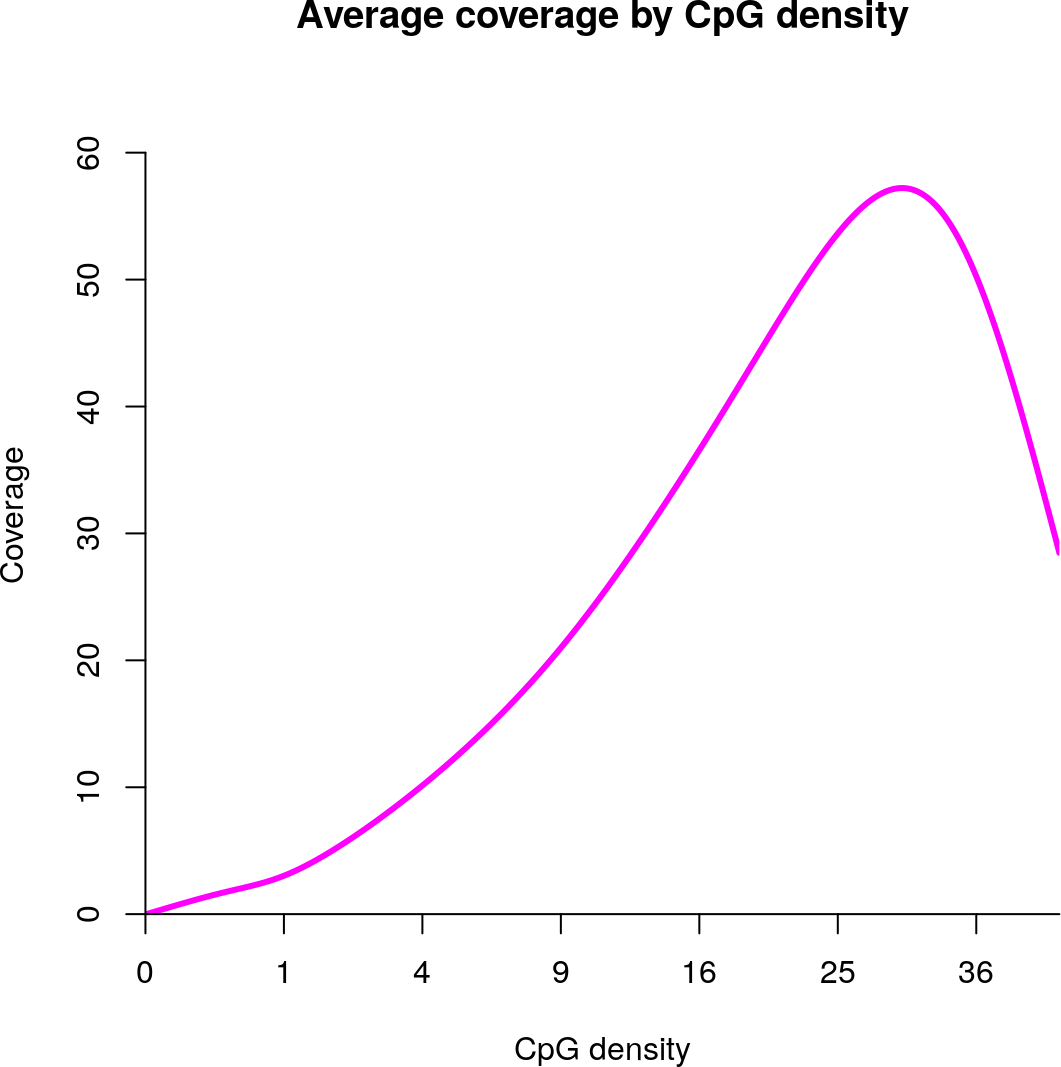Note. If a BAM file has previously been scanned, it will not be scanned again in subsequent calls of ramwas1scanBams. This way Step 1 can be rerun multiple times to efficiently include additional BAMs (samples) when they become available. Note. The BAM files are no longer needed after this step and the RaMWAS BAM info files are 50 to 100 times smaller than the original BAMs. ## 3.2 Summarize QC measures This step aggregates quality control metrics across all scanned BAM files, produces a number of summary plots and tables, and estimates fragment size distribution. In practice, multiple BAM files may correspond to the same sample. For simplicity, in the example here each BAM corresponds to one sample with the same name. RaMWAS can be instructed about BAM to sample correspondence via filebam2sample or bam2sample parameters (See parameter vignette). ramwas2collectqc(param) The following files and directories are created in the project directory: • Fragment_size_distribution.txt – text file with estimated fragment size distribution. • qc/Fragment_size_distribution_estimate.pdf – plot showing both estimated fragment size distribution and the distribution of distances from read starts to isolated CpGs. • Four sub-directories in the “qc” directory • summary_bams – by BAMs. This information can be used to exclude BAMs that fail selected QC criteria. • summary_bams_in_bam2sample – by BAMs, but only those listed in filebam2sample parameter. This information can be used to confirm that all BAMs that did not pass QC are no longer used in further analyses. • summary_by_sample – by sample. Slightly different QC criteria can be applied on sample level. For example, samples with low number of total reads can be excluded. • summary_total – total across all BAMs. This information presents the project summary of all BAMs in filebam2sample. • The directories listed above contain • Summary_QC.txt – table with a number of numerical QC measures, in an Excel friendly format. • Summary_QC_R.txt – table with a number of numerical QC measures, in an R friendly format. • qclist.rds – an R file with list of QC objects. • Fig_hist_*.pdf – histograms of several QC measures across samples. • Fig_*.pdf – PDF files with various QC plots, one page per BAM or sample, depending on the directory. After exclusion of BAMs and samples not passing QC, step 2 should be rerun. This ensures that fragment size distribution is estimated using selected data only. The fragment size distribution estimation plot is presented below. The points indicate the number of reads (y-axis) observed at varying distances from isolated CpGs (x-axis). The red line is the parametic fit for these points. For more information on estimation of fragment size distribution see (Oord et al. 2013). qc = readRDS(paste0(pfull$dirqc, "/summary_total/qclist.rds"))
frdata = qc$total$hist.isolated.dist1
con = paste0(pfull$dirproject,"/Fragment_size_distribution.txt"))) plotFragmentSizeDistributionEstimate(frdata, estimate)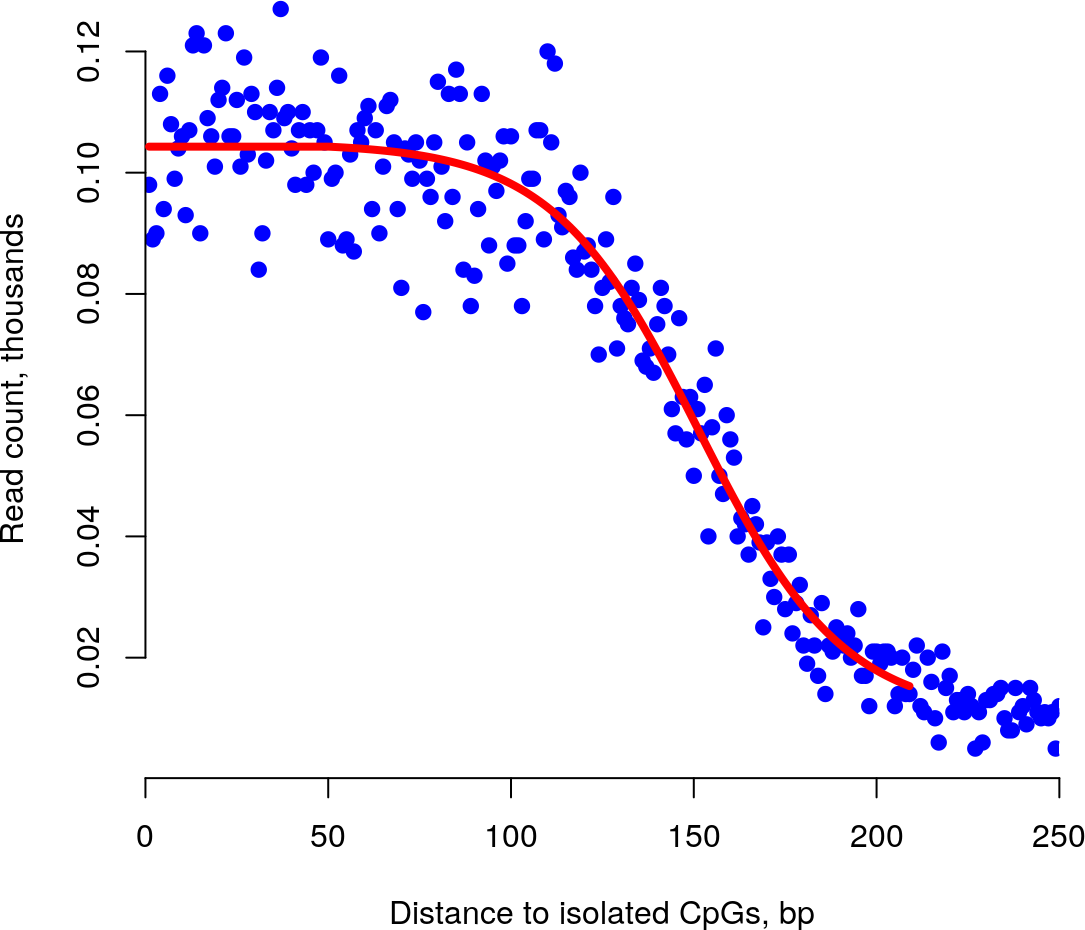## 3.3 Calculate CpG score matrix This step creates a CpG score matrix (a.k.a. fragment coverage matrix) for all samples and all CpGs in the CpG set. The samples are defined by either filebam2sample or bam2sample parameter. The CpGs are defined by the filecpgset parameter, see CpG set vignette for more information. RaMWAS can filter out CpGs with low scores. These CpGs are unmethylated and are unlikely to produce any findings. A CpG is preserved if • CpG has average equal or greater than the threshold minavgcpgcoverage (default is 0.3). • CpG has at least minnonzerosamples proportion of samples with nonzero coverage (default is 0.3, i.e. a CpG is preserved if at least 30% of samples have non-zero coverage). For each sample, the CpG scores are affected by the number of sequenced DNA fragments, which varies from sample to sample. To remove this variation, the CpG score matrix is normalized to have the same average score for each sample. ramwas3normalizedCoverage(param) This step a new creates directory named coverage_norm_X in the project directory (X is the number of samples, see Directory names) with the following files: • Coverage.* – filematrix with the CpG scores for all samples and all CpGs that passed the filtering. Each row has coverage for a single sample. Each column has coverage for a single CpG. See filematrix package for format details. • CpG_locations.* – filematrix with the location of the CpGs that passed the threshold. It has two columns – chr and position. • CpG_chromosome_names.txt – file with chromosome names (factor levels) for the integer column chr in CpG_locations.* filematrix. • raw_sample_sums.* – filematrix with total coverage for each sample before normalization. Note. This step created temporary files in the dirtemp directory. ## 3.4 Principal component analysis This step performs principal component analysis (PCA) on the CpG score matrix. PCA is capturing the main directions of unmeasured sources of variation in the data. The main goal of PCA is estimation of laboratory technical variations which can be used as covariates in the association analyses. PCA can be performed after regressing out known covariates as they represent measured sources of variation, which we need not estimate. Additionally, large sample loadings of the top PCs can indicate multidimensional outlying samples. Such sample may be excluded from the analysis. If measured covariates are regressed out prior to conducting the PCA, these covariates are loaded from the file filecovariates. The file can be comma or tab-separated, with sample names in the first column. The artificial data set includes a covariate file covariates.txt. The parameter modelcovariates names the covariates regressed out before PCA (NULL for none). ramwas4PCA(param) This step creates sub-directory PCA_X_cvrts_Y in the directory with score matrix, where X is the number of covariates regressed out and Y is a code distinguishing different sets with the same number of covariates (see Directory names). The sub-directory includes: • covmat.*, eigenvalues.*, and eigenvectors.* – filematrices with the sample covariance matrix and its eigenvalue decomposition. • PC_values.txt – principal components scores. • PC_loadings.txt – sample loadings for the top 20 PCs. • PC_plot_covariates_removed.pdf – plots of principal components scores (i.e. % variance explained on page 1) and sample loadings for the top 40 PCs (pages 2+). • PC_vs_covariates_corr.txt – correlations of principal components with phenotypes/covariates (from filecovariates file). • PC_vs_covariates_pvalue.txt – p-values for these correlations. The PC plot for artificial data shows one strong component and no outliers in the sample loadings, with first PC clearly capturing sample sex. eigenvalues = fm.load(paste0(pfull$dirpca, "/eigenvalues"));
eigenvectors = fm.open(
filenamebase = paste0(pfull$dirpca, "/eigenvectors"), readonly = TRUE); plotPCvalues(eigenvalues)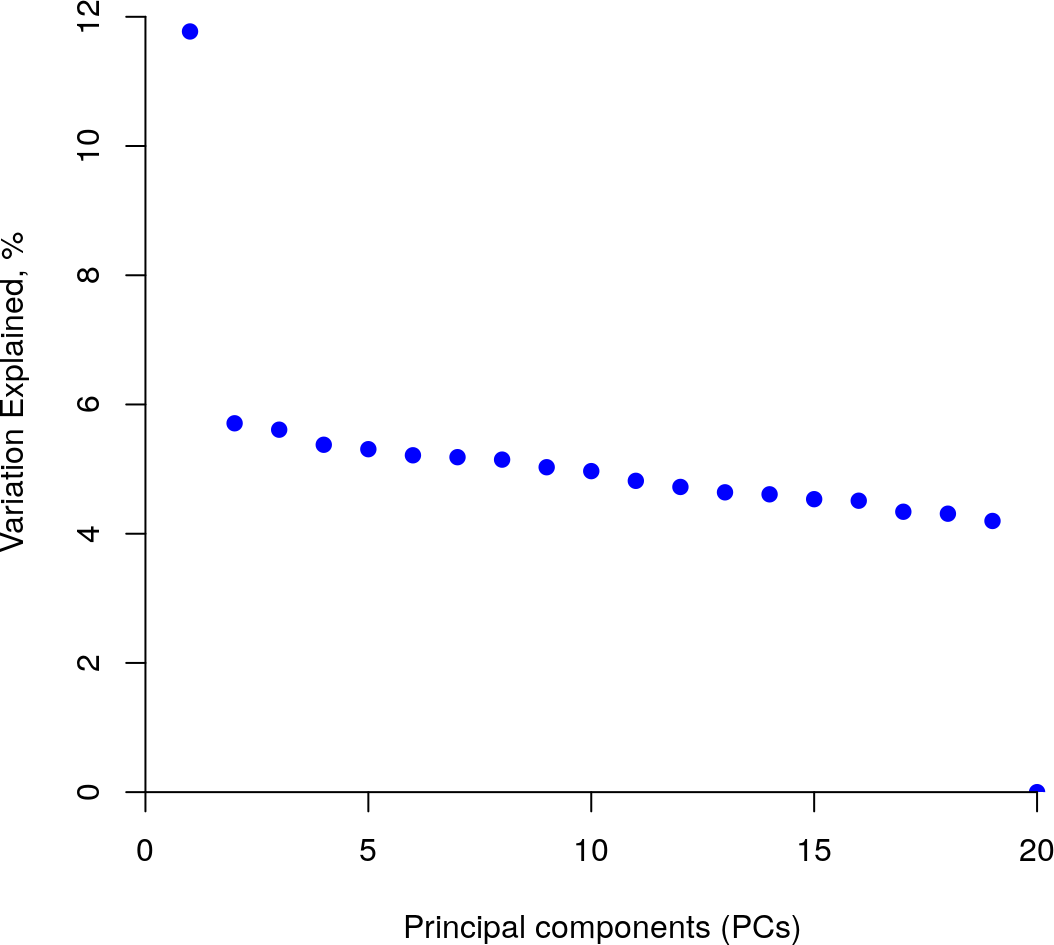plotPCvectors(eigenvectors[,1], 1)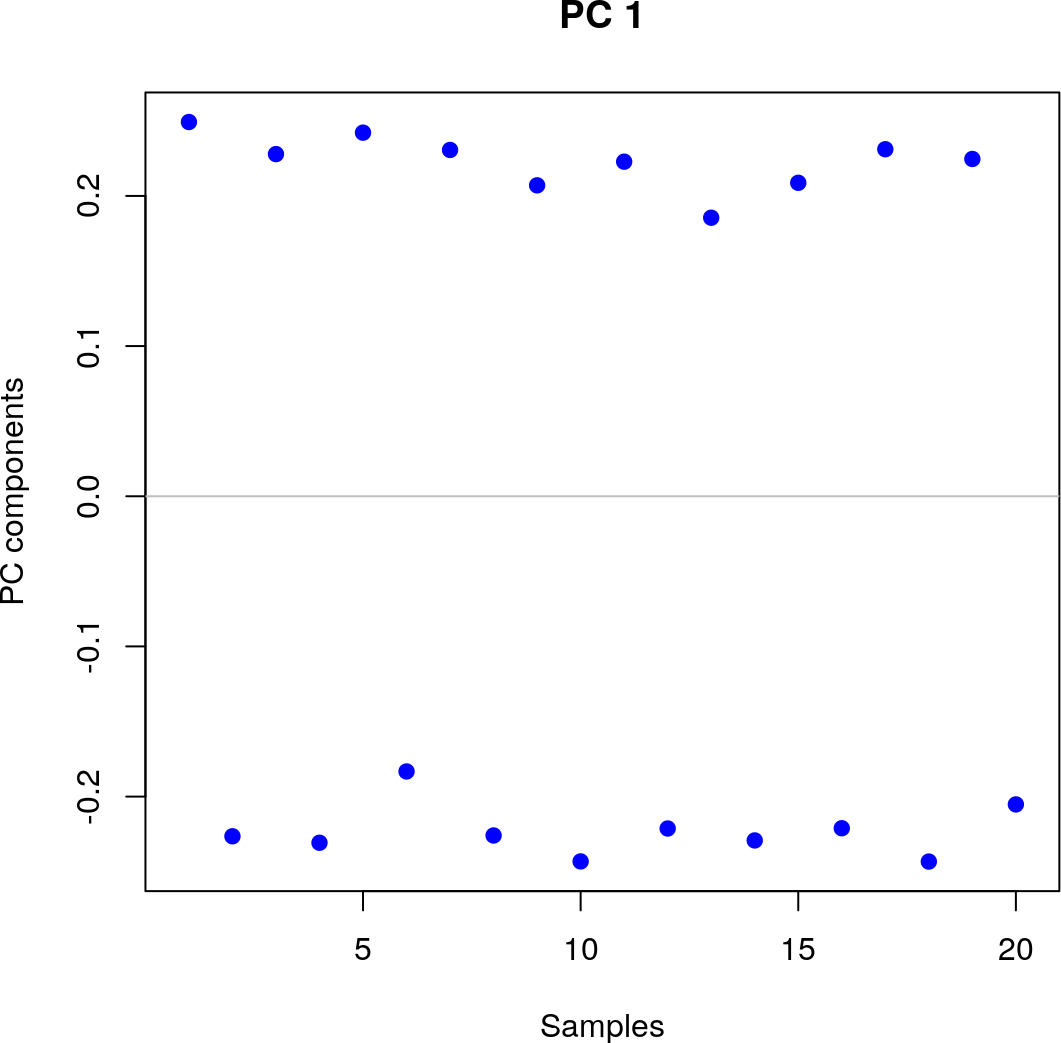plotPCvectors(eigenvectors[,2], 2)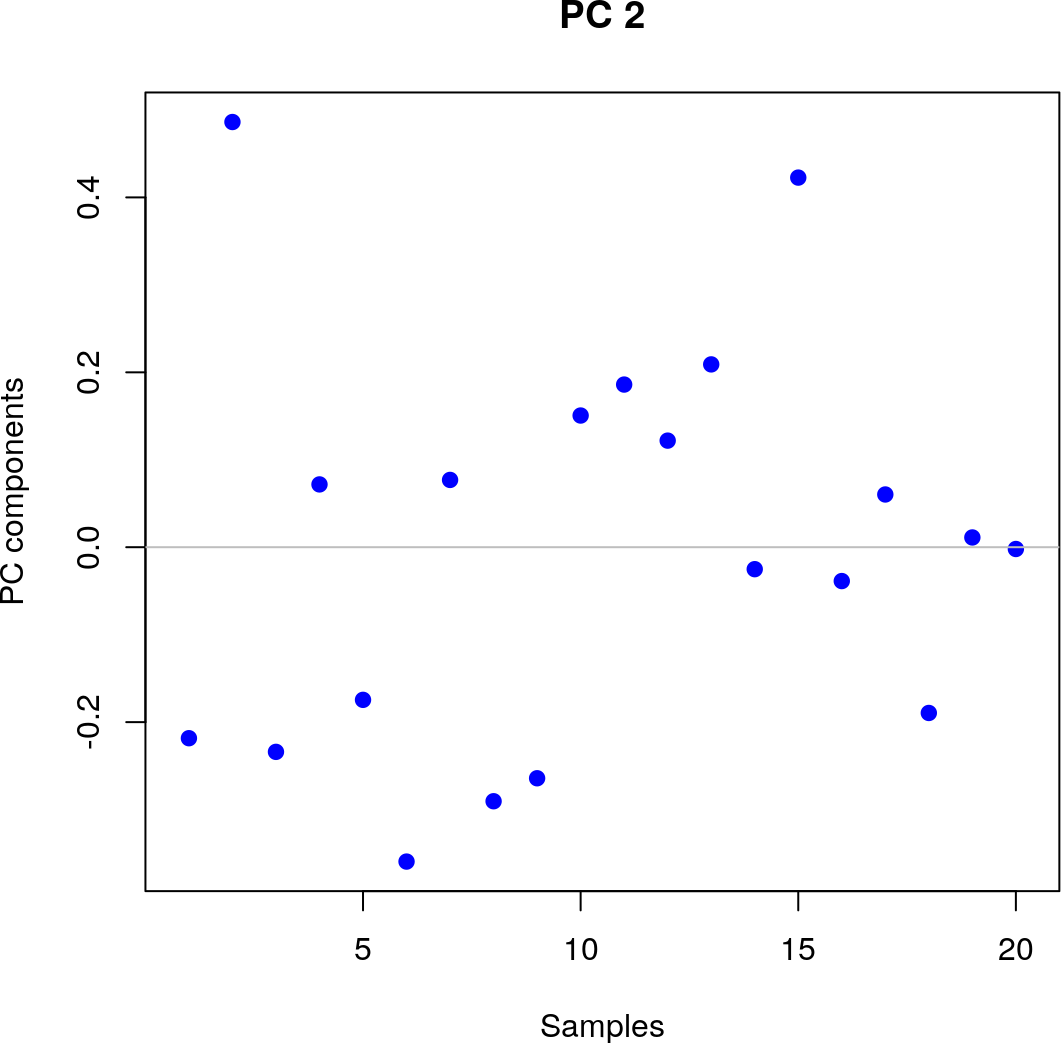close(eigenvectors) The file with correlations shows strong correlation of the top PCs with age and sex. tblcr = read.table( file = paste0(pfull$dirpca, "/PC_vs_covs_corr.txt"),
sep = "\t")
pander(head(tblcr, 3))
name age sex
PC1 -0.28 0.997
PC2 0.723 0.0335
PC3 0.184 -0.0281

The file with p-values indicate statistical significance of these correlations.

Table: Correlations in PC_vs_covariates_corr.txt file.

tblpv = read.table(
file = paste0(pfull\$dirpca, "/PC_vs_covs_pvalue.txt"),
sep = "\t")
pander(head(tblpv, 3))
P-values in PC_vs_covariates_pvalue.txt file.
name age sex
PC1 0.231 1.62e-21
PC2 0.000319 0.889
PC3 0.437 0.906

## 3.5 Methylome-wide association study (MWAS)

This step performs tests for association between normalized CpG scores and the outcome variable named by modeloutcome parameter. The analysis corrects for covariates listed in modelcovariates parameter and a number of top principal components (modelPCs). Tests are performed using the linear regression model if the outcome variable is numeric, ANOVA if categorical.

All results are saved in a filematrix. Findings passing the toppvthreshold p-value threshold are recorded in a text file.

ramwas5MWAS(param)

This step creates a sub-directory in the PCA directory named Testing_X_Y_PCs, where X is the name of the outcome variable and Y is the number of top PCs included as covariates (see Directory names). T